Do you like the article?
Share it with others —
Use new possibilities of MetaTrader 5

#### Similar articles# Developing a trading Expert Advisor from scratch (Part 10): Accessing custom indicators3 7990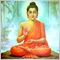### Introduction

A trading EA can be truly useful only if it can use custom indicators; otherwise, it is just a set of codes and instructions, which can be well designed, assist in managing positions or executing market deals, and that's probably all.

Well, the addition of indicators onto a MetaTrader 5 chart is not the hardest part. But accessing the data calculated by these indicators directly in the Expert Advisor, without proper planning, becomes an almost impossible task. And if we don't know how to do it, we are only limited to standard indicators. However, we need more for trading. A good example is the VWAP (Volume Weighted Average Price) indicator. It is a very important Moving Average for anyone trading futures on the Brazilian Stock Exchange. This MA is not presented among standard indicators in MetaTrader, but we can create a custom indicator that will calculate VWAP and display it on the screen. However, things get much more complicated when we decide to use the same indicator in a system that will be analyzed in the EA. Without the relevant knowledge, we won't be able to use this custom indicator inside an EA. In this article, we will see how to get around this limitation and solve this problem.

### Planning

First, let's try to create the calculations to use in our custom indicator. Fortunately, the VWAP calculation formula which we will use as an example, is quite simple.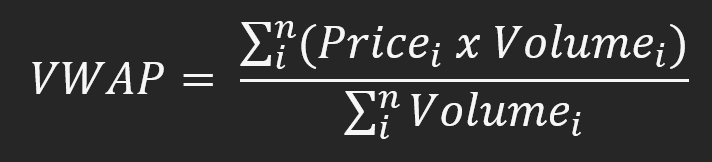When translated into a programming language, we get the following for MQL5:

```int OnCalculate(const int rates_total,
const int prev_calculated,
const datetime &time[],
const double &open[],
const double &high[],
const double &low[],
const double &close[],
const long &tick_volume[],
const long &volume[],
{
double          Price = 0;
ulong           Volume = 0;
static int      siPos = 0;

if (macroGetDate(time[rates_total - 1]) != macroGetDate(time[siPos]))
{
for (int c0 = rates_total - 1; macroGetDate(time[siPos]) != macroGetDate(time[c0]); siPos++);
ArrayInitialize(VWAP_Buff, EMPTY_VALUE);
}
for (int c0 = siPos; c0 < rates_total; c0++)
{
Price += ((high[c0] + low[c0] + close[c0]) / 3) * volume[c0];
Volume += volume[c0];
VWAP_Buff[c0] = Price / Volume;
}

return rates_total;
}

```

The line with the calculations is highlighted, while the rest of the function is used for a proper initialization of DAILY VWAP. However, our indicator still cannot be run on the chart, and we need to add a few more things to the code. The rest of the code can be seen below:

```#property copyright "Daniel Jose - Indicador VWAP ( IntraDay )"
#property version "1.01"
#property indicator_chart_window
#property indicator_buffers     1
#property indicator_plots       1
#property indicator_width1      2
#property indicator_type1 	DRAW_LINE
#property indicator_color1 	clrBlack
//+------------------------------------------------------------------+
#define macroGetDate(A) (A - (A % 86400))
//+------------------------------------------------------------------+
double VWAP_Buff[];
//+------------------------------------------------------------------+
int OnInit()
{
SetIndexBuffer(0, VWAP_Buff, INDICATOR_DATA);

return INIT_SUCCEEDED;
}

```

This enables the possibility of having VWAP on a chart as shown before: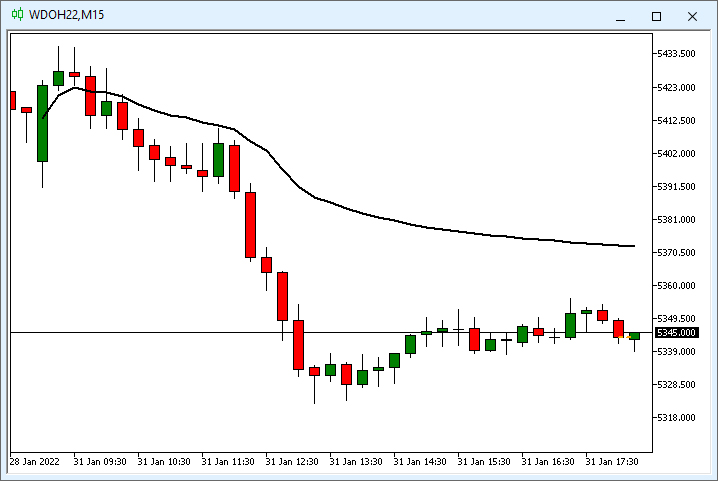Well, this part was not too much complicated. Now, we need to find a way to make the EA see VWAP, so that it analyzes the indicator in some specific way. This will make it possible to benefit from the indicator in trading.

For easier work with the indicator, let's save VWAP so that it can be easily accessed.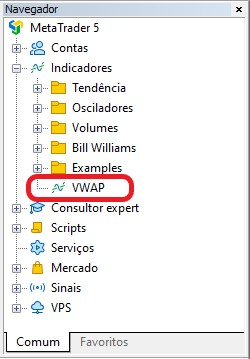After that, we can jump into a new way of projecting. Although the VWAP indicator is essentially correct, it is incorrectly programmed for use in an EA. Why? The problem is that the EA cannot know whether or not the indicator is on the chart. Without knowing this, it cannot read the indicator.

The problem is that file name matters little to the system. You can write any name in the file, but the indicator name should reflect what it is calculating. And our indicator does not yet have a name that reflects it. Even if it were called VWAP, it would mean nothing to the system. For this reason, the EA will not be able to know if the indicator is present on the chart or not.

To make the indicator reflect what it is calculating, we need to indicate this in code. This way we will create a unique name that will not necessarily be linked to the file name. In our case, the indicator initialization code should look like this:

```int OnInit()
{
SetIndexBuffer(0, VWAP_Buff, INDICATOR_DATA);
IndicatorSetString(INDICATOR_SHORTNAME, "VWAP");

return INIT_SUCCEEDED;
}

```

By simply adding the highlighted line we already solve the problem. In certain cases, it can be more difficult - we will get back to this later. First, let's use the code of the CUSTOM MOVING AVERAGE indicator from the MetaTrader 5 library as an example. Its code is as follows:

```void OnInit()
{
SetIndexBuffer(0,ExtLineBuffer,INDICATOR_DATA);
IndicatorSetInteger(INDICATOR_DIGITS,_Digits+1);
PlotIndexSetInteger(0,PLOT_DRAW_BEGIN,InpMAPeriod);
PlotIndexSetInteger(0,PLOT_SHIFT,InpMAShift);

string short_name;
switch(InpMAMethod)
{
case MODE_EMA :
short_name="EMA";
break;
case MODE_LWMA :
short_name="LWMA";
break;
case MODE_SMA :
short_name="SMA";
break;
case MODE_SMMA :
short_name="SMMA";
break;
default :
short_name="unknown ma";
}
IndicatorSetString(INDICATOR_SHORTNAME, short_name + "(" + string(InpMAPeriod) + ")");
PlotIndexSetDouble(0,PLOT_EMPTY_VALUE,0.0);
}

```

The highlighted part indicates the name we need. Note that it has nothing to do with the file name. But this must be done exactly inside the custom indicator.

Now that we have done that and made sure the EA will be able to check if the custom indicator is running on the chart or not, we can move on to the next step.

### Accessing the indicator through the EA

We can continue to do the way we did earlier. But ideally, to really understand what's going on, you should create completely new code. Since the idea is to learn to develop a trading Expert Advisor from scratch, let's go through this stage. Therefore, in the continuation of our journey, we will create an isolated Expert Advisor. Then we can include it or not into the final code. Now let's proceed to writing the code. The EA starts with the clean code, as you can see below:

```//+------------------------------------------------------------------+
int OnInit()
{
EventSetTimer(1);
return INIT_SUCCEEDED;
}
//+------------------------------------------------------------------+
void OnDeinit(const int reason)
{
EventKillTimer();
}
//+------------------------------------------------------------------+
void OnTick(){}
//+------------------------------------------------------------------+
void OnTimer(){}
//+------------------------------------------------------------------+

```

Let's do the following: first, we will assume that the VWAP indicator is on the chart and will load the last value calculated by the indicator into the Expert Advisor. We will repeat this every second. But how to do it? It's simple. See what the EA code looks like after the change:

```#property copyright "Daniel Jose"
#property version   "1.00"
//+------------------------------------------------------------------+
int     handle;
double  Buff[];
//+------------------------------------------------------------------+
int OnInit()
{
handle = ChartIndicatorGet(ChartID(), 0, "VWAP");
SetIndexBuffer(0, Buff, INDICATOR_DATA);

EventSetTimer(1);
return INIT_SUCCEEDED;
}
//+------------------------------------------------------------------+
void OnDeinit(const int reason)
{
EventKillTimer();
}
//+------------------------------------------------------------------+
void OnTick()
{
}
//+------------------------------------------------------------------+
void OnTimer()
{
int i;

if (handle != INVALID_HANDLE)
{
i = CopyBuffer(handle, 0, 0, 1, Buff);
Print(Buff);
}
}
//+------------------------------------------------------------------+

```

The highlighted parts are those that we have added to the clean code. The result is as follows: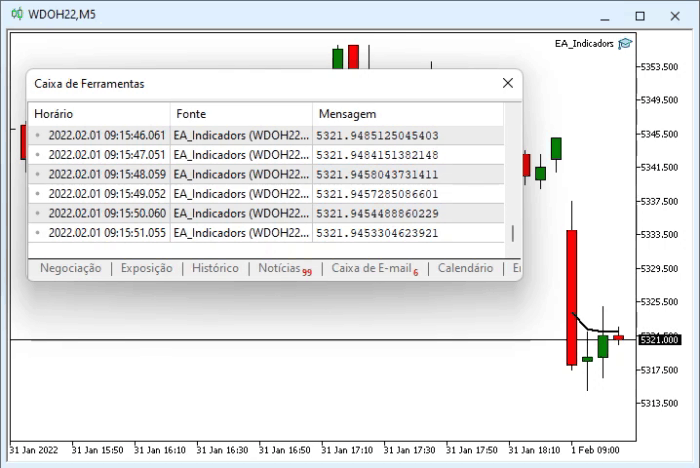Why did it work? This is because MQL5 provides means to read and write data between systems. One of the ways to read is to use the CopyBuffer function. It works like below: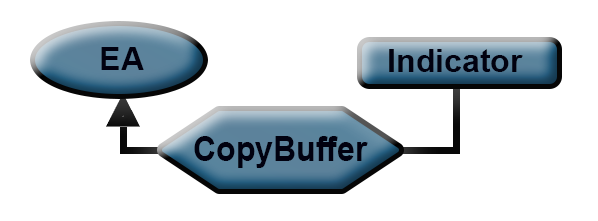Thus, we can read data from any custom indicator, i.e., we are not limited to standard MetaTrader 5 indicators. It means that we can create any indicator and it will work.

Now consider another scenario. This time VWAP does not exist on the chart. But the EA needs it, and we therefore need to load it onto the chart. How to do that? It's pretty simple too. Moreover, we have already used it before for other purposes - when creating a subwindow for the Expert Advisor. What we will do now is use the iCustom function. But this time we will load a custom indicator. Then the EA code will be like this:

```#property copyright "Daniel Jose"
#property version   "1.00"
//+------------------------------------------------------------------+
int     handle;
double  Buff[];
//+------------------------------------------------------------------+
int OnInit()
{
handle = ChartIndicatorGet(ChartID(), 0, "VWAP");
SetIndexBuffer(0, Buff, INDICATOR_DATA);

EventSetTimer(1);
return INIT_SUCCEEDED;
}
//+------------------------------------------------------------------+
void OnDeinit(const int reason)
{
EventKillTimer();
}
//+------------------------------------------------------------------+
void OnTick()
{
}
//+------------------------------------------------------------------+
void OnTimer()
{
int i;

if (handle == INVALID_HANDLE) handle = iCustom(NULL, PERIOD_CURRENT, "VWAP.EX5");else
{
i = CopyBuffer(handle, 0, 0, 1, Buff);
Print(Buff);
}
}
//+------------------------------------------------------------------+

```

The highlighted code is the only addition we have made to the original system. Running the EA now produces the following result: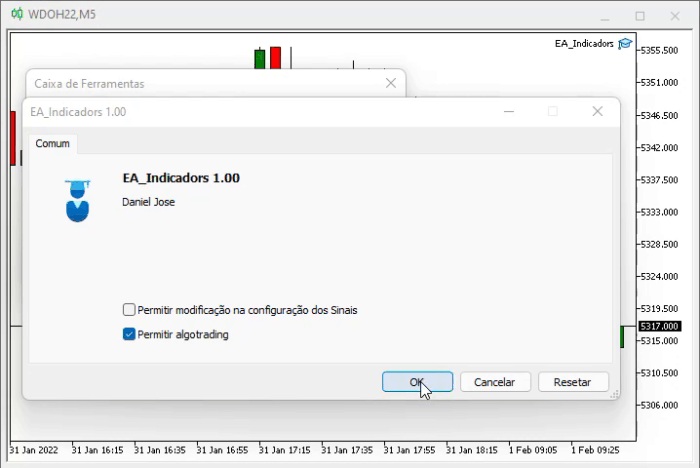The figure below shows what we have implemented: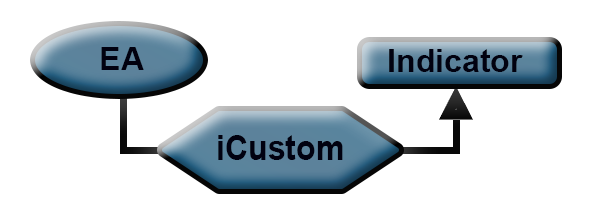That's all you need at the most basic level. But if you look closely, you will notice that VWAP is not visible on the chart. Even if the EA uses it, the user does not know what is going on. This can also be easily fixed, and the final code looks like the one below. Remember this: it's always good to be able to analyze and observe what the EA is doing, because it's not very safe to give it complete freedom, so I would not recommend doing so:

```#property copyright "Daniel Jose"
#property version   "1.00"
//+------------------------------------------------------------------+
int     handle;
long    id;
double  Buff[];
string  szCmd;
//+------------------------------------------------------------------+
int OnInit()
{
szCmd = "VWAP";
handle = ChartIndicatorGet(id = ChartID(), 0, szCmd);
SetIndexBuffer(0, Buff, INDICATOR_DATA);

EventSetTimer(1);
return INIT_SUCCEEDED;
}
//+------------------------------------------------------------------+
void OnDeinit(const int reason)
{
ChartIndicatorDelete(id, 0, szCmd);
IndicatorRelease(handle);
EventKillTimer();
}
//+------------------------------------------------------------------+
void OnTick()
{
}
//+------------------------------------------------------------------+
void OnTimer()
{
int i;

if (handle == INVALID_HANDLE)
{
if ((handle = iCustom(NULL, PERIOD_CURRENT, "VWAP.EX5")) != INVALID_HANDLE)
}else
{
i = CopyBuffer(handle, 0, 0, 1, Buff);
Print(Buff);
}
}
//+------------------------------------------------------------------+

```

The above EA code will read the last value calculated by VWAP, and will display it on the screen. It the indicator is not on the chart, it will be loaded and displayed. If we remove the EA form the chart, VWAP will also be removed from the screen. Thus, the EA will always have the things it needs to perform calculations. The results of what I have explained are shown below: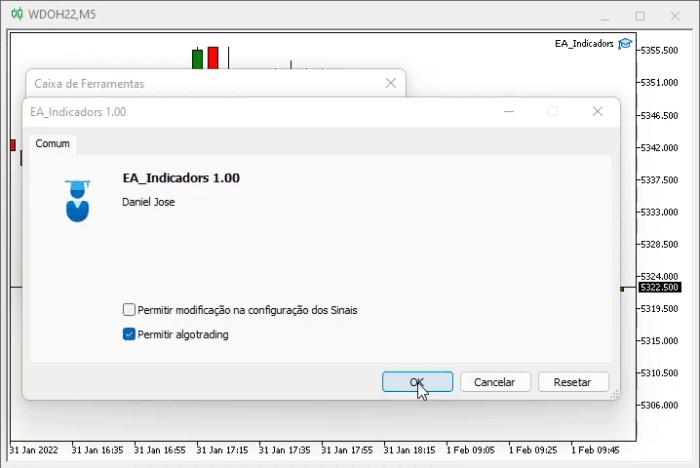One might think that this is not very feasible, since obviously we have not made any changes to the indicator. But even with the steps above, we can implement anything related to custom indicators. For a final explanation, let us consider another example. Let's apply a moving average and use the Expert Advisor in the same way as we did with VWAP, only now we will specify the parameters for the average.

### Second case: using moving averages

Calculation of the moving average is not important here, as we will focus on how to pass parameters into a custom indicator. Here is the new custom indicator:

```#property copyright "Daniel Jose 16.05.2021"
#property description "Basic Moving Averages (Optimizes Calculation)"
#property indicator_chart_window
//+------------------------------------------------------------------+
enum eTypeMedia
{
MME,    //Exponential moving average
MMA     //Arithmetic moving average
};
//+------------------------------------------------------------------+
#property indicator_buffers             1
#property indicator_plots               1
#property indicator_type1               DRAW_LINE
#property indicator_width1              2
#property indicator_applied_price       PRICE_CLOSE
//+------------------------------------------------------------------+
input color      user00 = clrRoyalBlue; //Cor
input int        user01 = 9;            //Periods
input eTypeMedia user02 = MME;          //MA type
input int user03 = 0;            //Displacement
//+------------------------------------------------------------------+
double Buff[], f_Expo;
//+------------------------------------------------------------------+
int OnInit()
{
string sz0 = "MM" + (user02 == MME ? "E": (user02 == MMA ? "A" : "_")) + (string)user01;

f_Expo = (double) (2.0 / (1.0 + user01));
ArrayInitialize(Buff, EMPTY_VALUE);
SetIndexBuffer(0, Buff, INDICATOR_DATA);
PlotIndexSetInteger(0, PLOT_LINE_COLOR, user00);
PlotIndexSetInteger(0, PLOT_DRAW_BEGIN, user01);
PlotIndexSetInteger(0, PLOT_SHIFT, user03);
IndicatorSetString(INDICATOR_SHORTNAME, sz0);

return INIT_SUCCEEDED;
}
//+------------------------------------------------------------------+
int OnCalculate(const int rates_total, const int prev_calculated, const int begin, const double &price[])
{
double Value;
int c0;

switch (user02)
{
case MME:
if (user01 < rates_total)
{
for (c0 = (prev_calculated > 0 ? prev_calculated - 1 : 0); c0 < rates_total - user03; c0++)
Buff[c0] = (c0 > 0? ((price[c0] - Buff[c0 - 1]) * f_Expo) + Buff[c0 - 1] : price[c0] * f_Expo);
for (; c0 < rates_total; c0++)
Buff[c0] = EMPTY_VALUE;
}
break;
case MMA:
if (user01 < rates_total)
{
if (prev_calculated == 0)
{
Value = 0;
for (int c1 = 0; c1 < user01; c1++) Value += price[user01 - c1];
Buff[user01] = Value / user01;
}
for (c0 = (prev_calculated > 0 ? prev_calculated - 1 : user01 + 1); c0 < rates_total - user03; c0++)
Buff[c0] = ((Buff[c0 - 1] * user01) - price[c0 - user01] + price[c0]) / user01;
for (; c0 < rates_total; c0++)
Buff[c0] = EMPTY_VALUE;
}
break;
}

return rates_total;
}
//+------------------------------------------------------------------+

```

Now the indicator name will depend on several factors. Later we can make the EA check and adjust to each situation. For example, let's say our EA uses two moving averages and displays them on the chart. Pay attention to highlighted parts in the above code - the enable the EA, and the iCustom function in this case, to change and configure indicator parameters. This is important to understand in order to be able to implement it if needed. So, one of the averages is 17-period exponential MA, and the other one is 52-period arithmetic MA. The 17-period MA will be green, and the 52-period one will be red. The EA will see the indicator as a function in the following form:

Average (Color, Period, Type, Shift) so now the indicator is not a separate file but an EA function. This is very common in programming because we call a program with the relevant parameters to execute a certain task and in the end we get the result easier. But the question is: How do we get our EA to create and manage this scenario in the same way as we did with VWAP?

For this, we need to change the EA code. The full code of the new EA is shown below:

```#property copyright "Daniel Jose"
#property version   "1.00"
//+------------------------------------------------------------------+
long    id;
int     handle1, handle2;
double  Buff1[], Buff2[];
string  szCmd1, szCmd2;
//+------------------------------------------------------------------+
int OnInit()
{
szCmd1 = "MME17";
szCmd2 = "MMA52";
id = ChartID();
handle1 = ChartIndicatorGet(id, 0, szCmd1);
handle2 = ChartIndicatorGet(id, 0, szCmd2);
SetIndexBuffer(0, Buff1, INDICATOR_DATA);
SetIndexBuffer(0, Buff2, INDICATOR_DATA);

EventSetTimer(1);
return INIT_SUCCEEDED;
}
//+------------------------------------------------------------------+
void OnDeinit(const int reason)
{
ChartIndicatorDelete(id, 0, szCmd1);
ChartIndicatorDelete(id, 0, szCmd2);
IndicatorRelease(handle1);
IndicatorRelease(handle2);
EventKillTimer();
}
//+------------------------------------------------------------------+
void OnTick()
{
}
//+------------------------------------------------------------------+
void OnTimer()
{
int i1, i2;

if (handle1 == INVALID_HANDLE)
{
if ((handle1 = iCustom(NULL, PERIOD_CURRENT, "Media Movel.EX5", clrGreen, 17, 0)) != INVALID_HANDLE)
};
if (handle2 == INVALID_HANDLE)
{
if ((handle2 = iCustom(NULL, PERIOD_CURRENT, "Media Movel.EX5", clrRed, 52, 1)) != INVALID_HANDLE)
};
if ((handle1 != INVALID_HANDLE) && (handle2 != INVALID_HANDLE))
{
i1 = CopyBuffer(handle1, 0, 0, 1, Buff1);
i2 = CopyBuffer(handle2, 0, 0, 1, Buff2);
Print(Buff1, "<< --- >>", Buff2);
}
}
//+------------------------------------------------------------------+

```

And here is the result: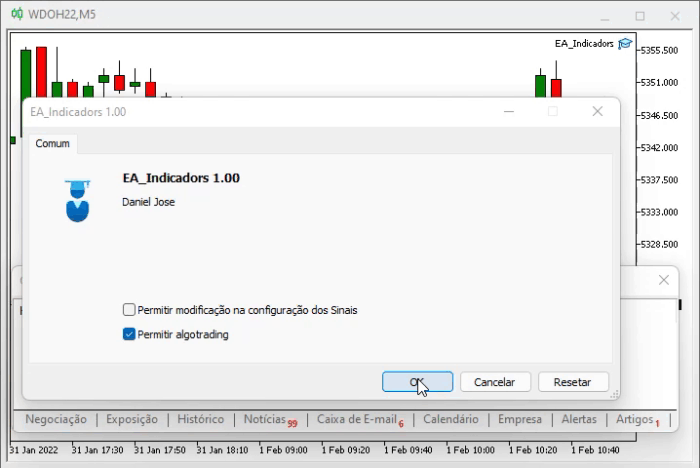Pay attention to the highlighted parts of the EA code. This is exactly what we need: we pass parameters to the indicator using the same mechanism that we used in VWAP. However, in the case of VWAP, there was no need to pass any parameters, in contrast to the moving averages, which do have parameters to be passed. All this provides a very large degree of freedom.

### Conclusion

This article does not contain universal code. Anyway, we went into detail about two different Expert Advisors and two different custom indicators, to understand how to use this kind of system in a more complex and thoughtful Expert Advisor. I believe that with this knowledge we can use our own custom indicators. Even our EA can provide a very interesting analysis. All of this proves that MetaTrader 5 is the most versatile platform a trader could wish for. If someone else has not understood this, then they simply have not studied it to the end.

Use the knowledge presented in this article, because MetaTrader 5 allows you to go much further than many have been able to do so far.

See you in the next article.

How to call indicators in MQL5

Custom indicators in MQL5 for beginners

MQL5 for Dummies: Guide to using technical indicator values in Expert Advisors

Translated from Portuguese by MetaQuotes Ltd.
Original article: https://www.mql5.com/pt/articles/10329

Attached files |

#### Other articles by this author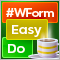DoEasy. Controls (Part 5): Base WinForms object, Panel control, AutoSize parameter
In the article, I will create the base object of all library WinForms objects and start implementing the AutoSize property of the Panel WinForms object — auto sizing for fitting the object internal content.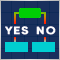Data Science and Machine Learning (Part 05): Decision Trees
Decision trees imitate the way humans think to classify data. Let's see how to build trees and use them to classify and predict some data. The main goal of the decision trees algorithm is to separate the data with impurity and into pure or close to nodes.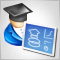Developing a trading Expert Advisor from scratch (Part 11): Cross order system
In this article we will create a system of cross orders. There is one type of assets that makes traders' life very difficult for traders — futures contracts. But why do they make life difficult?Developing a trading Expert Advisor from scratch (Part 9): A conceptual leap (II)
In this article, we will place Chart Trade in a floating window. In the previous part, we created a basic system which enables the use of templates within a floating window.